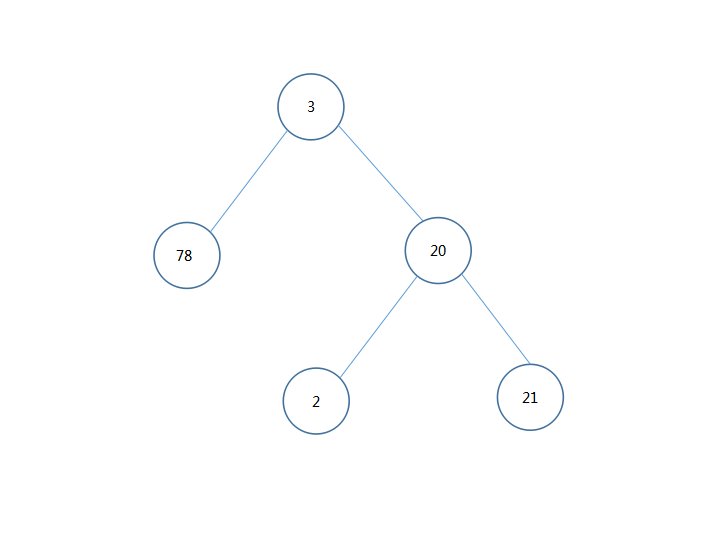38 二叉树遍历## 什么是二叉树？

class TreeNode:
def __init__(self, x):
self.val = x
self.left = None
self.right = None
public class TreeNode {
public int val;
public TreeNode left;
public TreeNode right;

public TreeNode(int value) {
this.val = value;
}
}

## 二叉树的遍历### 前序遍历 (Pre-Order Traversal)

class Solution(object):
def preorderTraversal(self, root):
"""
:type root: TreeNode
:rtype: List[int]
"""
res = []
if not root:
return res
res.append(root.val)
if root.left: # 左Node存在就按照按照相同的顺序（即先序遍历）取得root.left这棵子树中所有的value
res.extend(self.preorderTraversal(root.left))
if root.right: # 右Node存在就按照按照相同的顺序（即先序遍历）取得root.right这棵子树中所有的value
res.extend(self.preorderTraversal(root.right))
return res
class Solution {
List<Integer> list = new ArrayList<>();
public List<Integer> preorderTraversal(TreeNode root) {
// 递归终止条件，root 为 null 则遍历结束
if (root == null) {
return list;
}
// 前序遍历添加顺序：root--root.left--root.right
preorderTraversal(root.left);
preorderTraversal(root.right);
return list;
}
}

### 中序遍历 (In-Order Traversal)

class Solution(object):
def inorderTraversal(self, root):
"""
:type root: TreeNode
:rtype: List[int]
"""
res = []
if not root:
return res
if root.left: # 左Node存在就按照按照相同的顺序（即中序遍历）取得root.left这棵子树中所有的value
res.extend(self.inorderTraversal(root.left))
res.append(root.val)
if root.right: # 右Node存在就按照按照相同的顺序（即中序遍历）取得root.right这棵子树中所有的value
res.extend(self.inorderTraversal(root.right))
return res
class Solution {
List<Integer> list = new ArrayList<>();
public List<Integer> inorderTraversal(TreeNode root) {
// 递归终止条件，root 为 null 则遍历结束
if (root == null) {
return list;
}
// 中序遍历添加顺序：root.left--root--root.right
inorderTraversal(root.left);
inorderTraversal(root.right);
return list;
}
}

### 后序遍历 (Post-Order Traversal)

class Solution:
def postorderTraversal(self, root: TreeNode) -> List[int]:
res = []
if not root:
return res
if root.left: # 左Node存在就按照按照相同的顺序（即后序遍历）取得root.left这棵子树中所有的value
res.extend(self.postorderTraversal(root.left))
if root.right: # 右Node存在就按照按照相同的顺序（即右序遍历）取得root.right这棵子树中所有的value
res.extend(self.postorderTraversal(root.right))
res.append(root.val)
return res
class Solution {
List<Integer> list = new ArrayList<>();
public List<Integer> postorderTraversal(TreeNode root) {
// 递归终止条件，root 为 null 则遍历结束
if (root == null) {
return list;
}
// 后序遍历添加顺序：root.left--root.right--root
postorderTraversal(root.left);
postorderTraversal(root.right);
return list;
}
}

### 层序遍历 (Level-Order Traversal)

class Solution:
def levelOrder(self, root):
"""
:type root: TreeNode
:rtype: List[List[int]]
"""
def dfs(node, level, res):
if not node:
return
if len(res) < level: # 如果该层还未初始化
res.append([])
res[level-1].append(node.val) # 当前node属于第level层
dfs(node.left, level+1, res) # node.left属于第level+1层
dfs(node.right, level+1, res) # node.right属于第level+1层

res = []
dfs(root, 1, res)
return res

class Solution {
public List<List<Integer>> levelOrder(TreeNode root) {
List<List<Integer>> res = new ArrayList<>();
dfs(res, root, 0);
return res;
}

private void dfs(List<List<Integer>> res, TreeNode root, int level) {
// 递归终止条件，root 为 null 则遍历结束
if (root == null) {
return;
}
// 根据 level 以及集合的大小判断是否需要加入新的层级，也就是新的的 List
if (level >= res.size()) {
}
// 向下一个 level 中的左树进行遍历
dfs(res,root.left,level+1);
// 向下一个 level 中的左树进行遍历
dfs(res,root.right,level+1);
}
}

## 总结

• 树就是许许多多个Node组成的一个结构，要求这个结构联通且无环；
• Node就是一个节点，这个节点有两大属性，一个是 value，另一个就是它的子 Node；
• 对于二叉树来说，它的子 Node 最多有两个，因此二叉树有三个属性：value、left和right；
• 二叉树的遍历方式：
• 前序遍历
• 中序遍历
• 后序遍历
• 层序遍历